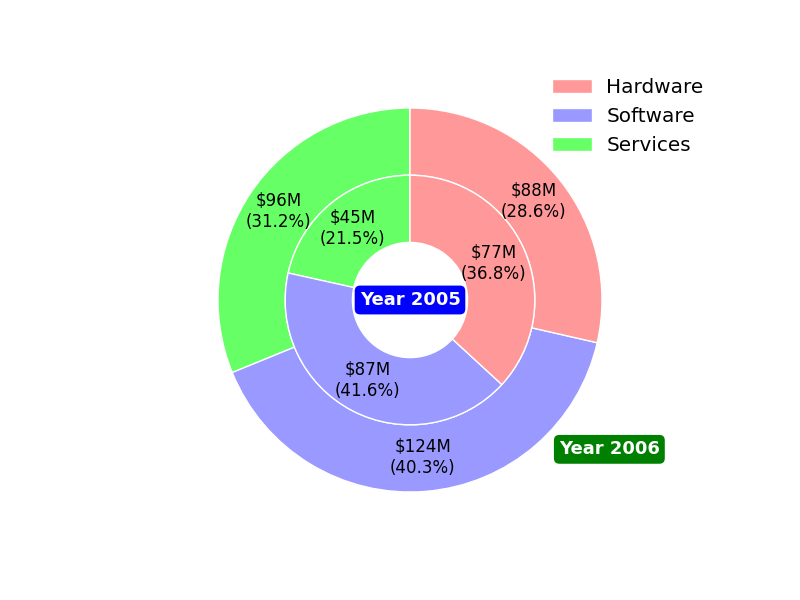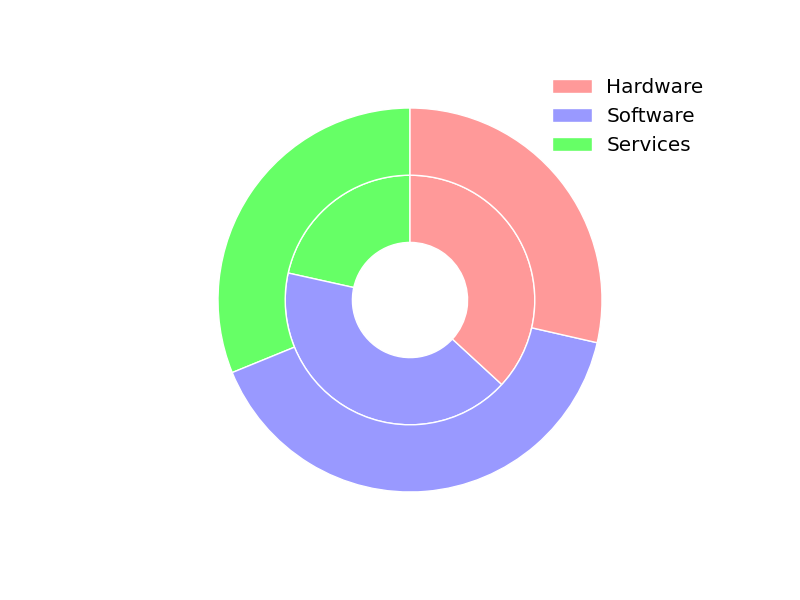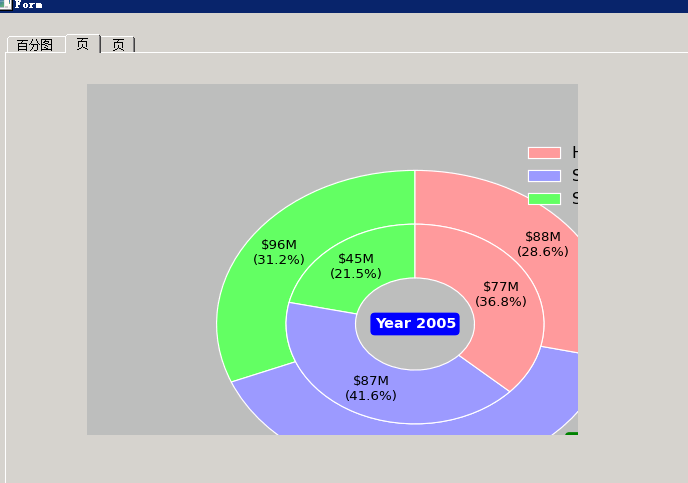# how to draw concentric donuts chart ?

Dear all

how to use matplotlib to drew chart like this ?

fwiw,
Alan Isaac

···

On 5/16/2014 7:51 AM, 不坏阿峰 wrote:

how to use matplotlib to drew chart like this ?

Alan is quite right. However, that aside, here's how you'd do it in
matplotlib:

import matplotlib.pyplot as plt

fig, ax = plt.subplots()
ax.axis('equal')

# Width of the "rings" (percentages if the largest "radius"==1)
width = 0.35

# Note the different "radius" values: largest --> outside "donut".
kwargs = dict(colors=['#66FF66', '#9999FF', '#FF9999'], startangle=90)
inside, _ = ax.pie([45, 87, 77], radius=1-width, **kwargs)
outside, _ = ax.pie([96, 124, 88], radius=1, **kwargs)

# This is the key. We'll set the "width" for all wedges generated by ax.pie.
# (The inside radius for each donut will be "radius" - "width")
plt.setp(inside + outside, width=width, edgecolor='white')

ax.legend(inside[::-1], ['Hardware', 'Software', 'Services'], frameon=False)

plt.show()

If you wanted to replicate the example figure more closely, you'll need to
get a touch fancier:

import matplotlib.pyplot as plt
import numpy as np

def pie(ax, values, **kwargs):
total = sum(values)
def formatter(pct):
return '\${:0.0f}M\n({:0.1f}%)'.format(pct*total/100, pct)
wedges, _, labels = ax.pie(values, autopct=formatter, **kwargs)
return wedges

fig, ax = plt.subplots()
ax.axis('equal')

width = 0.35
kwargs = dict(colors=['#66FF66', '#9999FF', '#FF9999'], startangle=90)

outside = pie(ax, [96, 124, 88], radius=1, pctdistance=1-width/2, **kwargs)
inside = pie(ax, [45, 87, 77], radius=1-width,
pctdistance=1 - (width/2) / (1-width), **kwargs)
plt.setp(inside + outside, width=width, edgecolor='white')

ax.legend(inside[::-1], ['Hardware', 'Software', 'Services'], frameon=False)

kwargs = dict(size=13, color='white', va='center', fontweight='bold')
ax.text(0, 0, 'Year 2005', ha='center',
bbox=dict(boxstyle='round', facecolor='blue', edgecolor='none'),
**kwargs)
ax.annotate('Year 2006', (0, 0), xytext=(np.radians(-45), 1.1),
bbox=dict(boxstyle='round', facecolor='green',
edgecolor='none'),
textcoords='polar', ha='left', **kwargs)

plt.show()

Hope those examples give you some ideas!
Cheers,
-Joe···

On Fri, May 16, 2014 at 7:36 AM, Alan G Isaac <alan.isaac@...287...> wrote:

On 5/16/2014 7:51 AM, 不坏阿峰 wrote:
> how to use matplotlib to drew chart like this ?

fwiw,
Alan Isaac

very nice and thanks a lot , you are very nice.
and could you give me some guide how to put this in qlabel of pyqt ? or what’s widget is better to work with these code···

2014-05-16 21:25 GMT+07:00 Joe Kington <joferkington@…287…>:

Instantly run your Selenium tests across 300+ browser/OS combos.

Get unparalleled scalability from the best Selenium testing platform available

http://p.sf.net/sfu/SauceLabs

Matplotlib-users mailing list

Matplotlib-users@lists.sourceforge.net

https://lists.sourceforge.net/lists/listinfo/matplotlib-users

On Fri, May 16, 2014 at 7:36 AM, Alan G Isaac <alan.isaac@…287…> wrote:

On 5/16/2014 7:51 AM, 不坏阿峰 wrote:

how to use matplotlib to drew chart like this ?

fwiw,

Alan Isaac

Alan is quite right. However, that aside, here’s how you’d do it in matplotlib:

import matplotlib.pyplot as plt

fig, ax = plt.subplots()
ax.axis(‘equal’)

width = 0.35

# Note the different “radius” values: largest --> outside “donut”.

kwargs = dict(colors=[’#66FF66’, ‘#9999FF’, ‘#FF9999’], startangle=90)

inside, _ = ax.pie([45, 87, 77], radius=1-width, **kwargs)
outside, _ = ax.pie([96, 124, 88], radius=1, **kwargs)

# (The inside radius for each donut will be “radius” - “width”)

plt.setp(inside + outside, width=width, edgecolor=‘white’)

ax.legend(inside[::-1], [‘Hardware’, ‘Software’, ‘Services’], frameon=False)

plt.show()

If you wanted to replicate the example figure more closely, you’ll need to get a touch fancier:

import matplotlib.pyplot as plt

import numpy as np

def pie(ax, values, **kwargs):
total = sum(values)
def formatter(pct):
return ‘\${:0.0f}M\n({:0.1f}%)’.format(pct*total/100, pct)
wedges, _, labels = ax.pie(values, autopct=formatter, **kwargs)

``````return wedges
``````

fig, ax = plt.subplots()
ax.axis(‘equal’)

width = 0.35
kwargs = dict(colors=[’#66FF66’, ‘#9999FF’, ‘#FF9999’], startangle=90)

outside = pie(ax, [96, 124, 88], radius=1, pctdistance=1-width/2, **kwargs)

inside = pie(ax, [45, 87, 77], radius=1-width,
pctdistance=1 - (width/2) / (1-width), **kwargs)
plt.setp(inside + outside, width=width, edgecolor=‘white’)

ax.legend(inside[::-1], [‘Hardware’, ‘Software’, ‘Services’], frameon=False)

kwargs = dict(size=13, color=‘white’, va=‘center’, fontweight=‘bold’)
ax.text(0, 0, ‘Year 2005’, ha=‘center’,
bbox=dict(boxstyle=‘round’, facecolor=‘blue’, edgecolor=‘none’),

``````    **kwargs)
``````

ax.annotate(‘Year 2006’, (0, 0), xytext=(np.radians(-45), 1.1),
bbox=dict(boxstyle=‘round’, facecolor=‘green’, edgecolor=‘none’),
textcoords=‘polar’, ha=‘left’, **kwargs)

plt.show()

Hope those examples give you some ideas!

Cheers,

-Joe

i have try modify Official example use my Qwidget in my Ui. it works. i am fresher to use matplotlib , maybe my question is stupid . your code is use pyplot, is the same way to use like below code? i do not how to change your code to a class, and use with Qwidget.

hope you can give me some guide. thanks a lot···

2014-05-16 22:23 GMT+07:00 不坏阿峰 <onlydebian@…287…>:

very nice and thanks a lot , you are very nice.
and could you give me some guide how to put this in qlabel of pyqt ? or what’s widget is better to work with these code

2014-05-16 21:25 GMT+07:00 Joe Kington <joferkington@…287…>:

Instantly run your Selenium tests across 300+ browser/OS combos.

Get unparalleled scalability from the best Selenium testing platform available

http://p.sf.net/sfu/SauceLabs

Matplotlib-users mailing list

Matplotlib-users@lists.sourceforge.net

https://lists.sourceforge.net/lists/listinfo/matplotlib-users

On Fri, May 16, 2014 at 7:36 AM, Alan G Isaac <alan.isaac@…287…> wrote:

On 5/16/2014 7:51 AM, 不坏阿峰 wrote:

how to use matplotlib to drew chart like this ?

fwiw,

Alan Isaac

Alan is quite right. However, that aside, here’s how you’d do it in matplotlib:

import matplotlib.pyplot as plt

fig, ax = plt.subplots()
ax.axis(‘equal’)

width = 0.35

# Note the different “radius” values: largest --> outside “donut”.

kwargs = dict(colors=[’#66FF66’, ‘#9999FF’, ‘#FF9999’], startangle=90)

inside, _ = ax.pie([45, 87, 77], radius=1-width, **kwargs)
outside, _ = ax.pie([96, 124, 88], radius=1, **kwargs)

# (The inside radius for each donut will be “radius” - “width”)

plt.setp(inside + outside, width=width, edgecolor=‘white’)

ax.legend(inside[::-1], [‘Hardware’, ‘Software’, ‘Services’], frameon=False)

plt.show()

If you wanted to replicate the example figure more closely, you’ll need to get a touch fancier:

import matplotlib.pyplot as plt

import numpy as np

def pie(ax, values, **kwargs):
total = sum(values)
def formatter(pct):
return ‘\${:0.0f}M\n({:0.1f}%)’.format(pct*total/100, pct)
wedges, _, labels = ax.pie(values, autopct=formatter, **kwargs)

``````return wedges
``````

fig, ax = plt.subplots()
ax.axis(‘equal’)

width = 0.35
kwargs = dict(colors=[’#66FF66’, ‘#9999FF’, ‘#FF9999’], startangle=90)

outside = pie(ax, [96, 124, 88], radius=1, pctdistance=1-width/2, **kwargs)

inside = pie(ax, [45, 87, 77], radius=1-width,
pctdistance=1 - (width/2) / (1-width), **kwargs)
plt.setp(inside + outside, width=width, edgecolor=‘white’)

ax.legend(inside[::-1], [‘Hardware’, ‘Software’, ‘Services’], frameon=False)

kwargs = dict(size=13, color=‘white’, va=‘center’, fontweight=‘bold’)
ax.text(0, 0, ‘Year 2005’, ha=‘center’,
bbox=dict(boxstyle=‘round’, facecolor=‘blue’, edgecolor=‘none’),

``````    **kwargs)
``````

ax.annotate(‘Year 2006’, (0, 0), xytext=(np.radians(-45), 1.1),
bbox=dict(boxstyle=‘round’, facecolor=‘green’, edgecolor=‘none’),
textcoords=‘polar’, ha=‘left’, **kwargs)

plt.show()

Hope those examples give you some ideas!

Cheers,

-Joe

Dear all

i try do some modify, it can display the diagram, but not correct. could you help me correct it ? sorry that i knew a bit about matplotlib. many thanks!

only display like this.···

2014-05-17 11:39 GMT+07:00 不坏阿峰 <onlydebian@…878…287…>:

i have try modify Official example use my Qwidget in my Ui. it works. i am fresher to use matplotlib , maybe my question is stupid . your code is use pyplot, is the same way to use like below code? i do not how to change your code to a class, and use with Qwidget.

hope you can give me some guide. thanks a lot

from future import unicode_literals

import sys, os, random

from PyQt4 import QtGui, QtCore

from numpy import arange, sin, pi

from matplotlib.backends.backend_qt4agg import FigureCanvasQTAgg as FigureCanvas

from matplotlib.figure import Figure

from mychart_ui import Ui_Form

class MyMplCanvas(FigureCanvas):

“”“Ultimately, this is a QWidget (as well as a FigureCanvasAgg, etc.).”""

def init(self, parent=None, width=5, height=4, dpi=100):

fig = Figure(figsize=(width, height), dpi=dpi)

# We want the axes cleared every time plot() is called

self.axes.hold(False)

self.compute_initial_figure()

FigureCanvas.init(self, fig)

self.setParent(parent)

FigureCanvas.setSizePolicy(self,

QtGui.QSizePolicy.Expanding,

QtGui.QSizePolicy.Expanding)

FigureCanvas.updateGeometry(self)

def compute_initial_figure(self):

pass

class MyStaticMplCanvas(MyMplCanvas):

“”“Simple canvas with a sine plot.”""

def compute_initial_figure(self):

t = arange(0.0, 3.0, 0.01)

s = sin(2pit)

self.axes.plot(t, s)

class myWidget(QtGui.QWidget, Ui_Form):

def init(self,parent=None):

QtGui.QWidget.init(self, parent)

self.setupUi(self)

sc = MyStaticMplCanvas(self.matwidget, width=5, height=4, dpi=100)

qApp = QtGui.QApplication(sys.argv)

aw = myWidget()

aw.show()

sys.exit(qApp.exec_())

<<<<<<<<

2014-05-16 22:23 GMT+07:00 不坏阿峰 <onlydebian@…287…>:

very nice and thanks a lot , you are very nice.
and could you give me some guide how to put this in qlabel of pyqt ? or what’s widget is better to work with these code

2014-05-16 21:25 GMT+07:00 Joe Kington <joferkington@…287…>:

Instantly run your Selenium tests across 300+ browser/OS combos.

Get unparalleled scalability from the best Selenium testing platform available

http://p.sf.net/sfu/SauceLabs

Matplotlib-users mailing list

Matplotlib-users@lists.sourceforge.net

https://lists.sourceforge.net/lists/listinfo/matplotlib-users

On Fri, May 16, 2014 at 7:36 AM, Alan G Isaac <alan.isaac@…287…> wrote:

On 5/16/2014 7:51 AM, 不坏阿峰 wrote:

how to use matplotlib to drew chart like this ?

fwiw,

Alan Isaac

Alan is quite right. However, that aside, here’s how you’d do it in matplotlib:

import matplotlib.pyplot as plt

fig, ax = plt.subplots()
ax.axis(‘equal’)

width = 0.35

# Note the different “radius” values: largest --> outside “donut”.

kwargs = dict(colors=[’#66FF66’, ‘#9999FF’, ‘#FF9999’], startangle=90)

inside, _ = ax.pie([45, 87, 77], radius=1-width, **kwargs)
outside, _ = ax.pie([96, 124, 88], radius=1, **kwargs)

# (The inside radius for each donut will be “radius” - “width”)

plt.setp(inside + outside, width=width, edgecolor=‘white’)

ax.legend(inside[::-1], [‘Hardware’, ‘Software’, ‘Services’], frameon=False)

plt.show()

If you wanted to replicate the example figure more closely, you’ll need to get a touch fancier:

import matplotlib.pyplot as plt

import numpy as np

def pie(ax, values, **kwargs):
total = sum(values)
def formatter(pct):
return ‘\${:0.0f}M\n({:0.1f}%)’.format(pct*total/100, pct)
wedges, _, labels = ax.pie(values, autopct=formatter, **kwargs)

``````return wedges
``````

fig, ax = plt.subplots()
ax.axis(‘equal’)

width = 0.35
kwargs = dict(colors=[’#66FF66’, ‘#9999FF’, ‘#FF9999’], startangle=90)

outside = pie(ax, [96, 124, 88], radius=1, pctdistance=1-width/2, **kwargs)

inside = pie(ax, [45, 87, 77], radius=1-width,
pctdistance=1 - (width/2) / (1-width), **kwargs)
plt.setp(inside + outside, width=width, edgecolor=‘white’)

ax.legend(inside[::-1], [‘Hardware’, ‘Software’, ‘Services’], frameon=False)

kwargs = dict(size=13, color=‘white’, va=‘center’, fontweight=‘bold’)
ax.text(0, 0, ‘Year 2005’, ha=‘center’,
bbox=dict(boxstyle=‘round’, facecolor=‘blue’, edgecolor=‘none’),

``````    **kwargs)
``````

ax.annotate(‘Year 2006’, (0, 0), xytext=(np.radians(-45), 1.1),
bbox=dict(boxstyle=‘round’, facecolor=‘green’, edgecolor=‘none’),
textcoords=‘polar’, ha=‘left’, **kwargs)

plt.show()

Hope those examples give you some ideas!

Cheers,

-Joe